Home | | Maths 12th Std | Shortest distance between two straight lines

# Shortest distance between two straight lines

We have just explained how the point of intersection of two lines are found and we have also studied how to determine whether the given two lines are parallel or not.

Shortest distance between two straight lines

We have just explained how the point of intersection of two lines are found and we have also studied how to determine whether the given two lines are parallel or not.

Definition 6.6

Two lines are said to be coplanar if they lie in the same plane.

Note

If two lines are either parallel or intersecting, then they are coplanar.

Definition 6.7

Two lines in space are called skew lines if they are not parallel and do not intersect

Note

If two lines are skew lines, then they are non coplanar. If the lines are not parallel and intersect, the distance between them is zero. If they are parallel and non-intersecting, the distance is determined by the length of the line segment perpendicular to both the parallel lines. In the same way, the shortest distance between two skew lines is defined as the length of the line segment perpendicular to both the skew lines. Two lines will either be parallel or skew.Proof

The given two parallel linessandtare denoted by L1 and L2 respectively. Let A and B be the points on L1 and L2 whose position vectors areandrespectively. The two given lines are parallel to.

Let AD be a perpendicular to the two given lines. If θ is the acute angle betweenand, thenBut, from the right angle triangle ABD ,Proof

The two skew lines=+ sand=+ tare denoted by L1 and L2 respectively.

Let A and C be the points on L1 and L2 with position vectorsandrespectively.

From the given equations of skew lines, we observe that L1 is parallel to the vectorand L2 is parallel to the vector. So,×is perpendicular to the lines L1 and L2.Let SD  be the line segment perpendicular to both the lines L1 and L2. Then the vectoris perpendicular to the vectorsandand therefore it is parallel to the vector×.

So,is a unit vector in the direction of. Then, the shortest distance || is the absolute value of the projection ofon. That is,

δ = || =|. (Unit vector in the direction of)|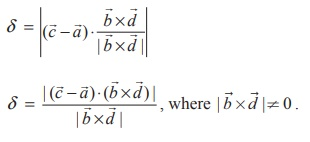Remark

(i) It follows from theorem (6.14) that two straight lines=+ sand=+ tintersect each other (that is, coplanar) if (-) . (×) = 0

(2) If two linesintersect each other  (that is, coplanar), then we have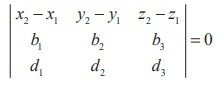Example 6.34

Find the parametric form of vector equation of a straight line passing through the point of intersection of the straight linesand perpendicular to both straight lines.

Solution

The Cartesian equations of the straight line= (iˆ + 3 ˆj k ) + t(2iˆ + 3 ˆj + 2k ) isThen any point on this line is of the form (2s +1, 3s + 3, 2s -1)          ... (1)

The Cartesian equation of the second line is (x – 2)/1 = (y – 4)/2 = (z + 3)/4 = t  (say)

Then any point on this line is of the form (t + 2, 2t + 4, 4t - 3)

If the given lines intersect, then there must be a common point. Therefore, for some s, t R, we have (2s +1, 3s + 3, 2s −1) = (t + 2, 2t + 4, 4t − 3) .

Equating the coordinates of x, y and z we get

2s t = 1, 3s − 2t = 1 and s − 2t = −1.

Solving the first two of the above three equations, we get s = 1 and t = 1. These values of s and t satisfy the third equation. So, the lines are intersecting.

Now, using the value of s in (1) or the value of t in (2), the point of intersection (3, 6,1) of these two straight lines is obtained.

If we take= 2iˆ + 3ˆj + 2kˆ  and= iˆ + 2ˆj + 4kˆ  thenis a vector perpendicular to both the given straight lines. Therefore, the required straight line passing through (3, 6,1) and perpendicular to both the given straight lines is the same as the straight line passing through (3, 6,1) and parallel to 8iˆ − 6 ˆj + kˆ . Thus, the equation of the required straight line isExample 6.35

Determine whether the pair of straight lines= (2ˆi + 6ˆj + 3ˆk ) + t(2ˆi + 3ˆj + 4ˆk ) ,= (2ˆj − 3ˆk ) + s(ˆi + 2ˆj + 3ˆk ) are parallel. Find the shortest distance between them.

Solution

Comparing the given two equations with=+ sand=+ twe have= 2ˆi + 6ˆj + 3ˆk,= 2ˆi + 3ˆj + 4ˆk,= 2ˆj − 3ˆk,= ˆi + 2ˆj + 3ˆk

Clearly,is not a scalar multiple of. So, the two vectors are not parallel and hence the two lines are not parallel.

The shortest distance between the two straight lines is given by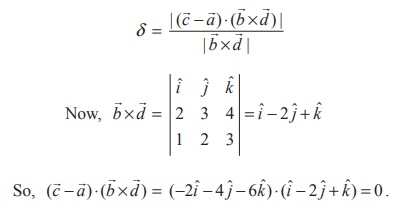Therefore, the distance between the two given straight lines is zero.Thus, the given lines intersect each other.

Example 6.36

Find the shortest distance between the two given straight lines= (2ˆi + 3ˆj + 4ˆk ) + t(−2ˆi + ˆj − 2ˆk ) andSolution

The parametric form of vector equations of the given straight lines areClearly,is a scalar multiple of, and hence the two straight lines are parallel. We know that the shortest distance between two parallel straight lines is given by d =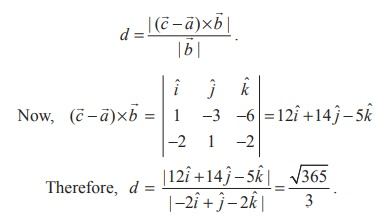Example 6.37

Find the coordinates of the foot of the perpendicular drawn from the point (−1, 2, 3) to the straight line= (ˆi − 4ˆj + 3ˆk ) + t(2ˆi + 3ˆj + ˆk ) . Also, find the shortest distance from the given point to the straight line.

Solution

Comparing the given equation= (ˆi - 4ˆj + 3ˆk ) + t(2ˆi + 3ˆj + ˆk ) with=+ t, we get a = ˆi - 4ˆj + 3ˆk , and= 2ˆi + 3ˆj + ˆk . We denote the given point (-1, 2, 3) by D , and the point (1, -4, 3) on the straight line by A . If F is the foot of the perpendicular from D to the straight line, then F is of the form (2t +1, 3t - 4, t + 3) and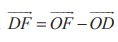= (2t + 2)iˆ + (3t - 6) ˆj + tkˆ.Sinceis perpendicular to, we have.= 0 2(2t + 2) + 3(3t - 6) +1(t) = 0

t = 1

Therefore, the coordinate of F is  (3,-1, 4)

Now, the perpendicular distance from the given point to the given line is

DF = ||= √[42+(-3)2+12] =  √26 units.

Tags : Definition, Theorem, Proof, Solved Example Problems, Solution , 12th Mathematics : UNIT 6 : Applications of Vector Algebra
Study Material, Lecturing Notes, Assignment, Reference, Wiki description explanation, brief detail
12th Mathematics : UNIT 6 : Applications of Vector Algebra : Shortest distance between two straight lines | Definition, Theorem, Proof, Solved Example Problems, Solution